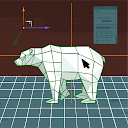5:22 AM
-3Let $\sum\limits_{k=1}^{\infty}\frac{2^{-k}}{1+2^{2^{-k}}} = q + \frac{p}{ln(d)}$ How to find $q, p, d$ ?

I have removed the tag from this question: math.stackexchange.com/posts/4110295/revisions
There are two more questions tagged :
0I am currently working on something that requires a method I can use to convert coordinates on an octagon to coordinates on a double Torus. The octagon is a regular octagon. Does anyone know of a way to do so? I am a beginner to this field so please be easy on me.

1Fix a continuous function f : [0, 1] × [0, 1] → R. For each t ∈ [0, 1] we define ft : [0,1] → R via ft(s) = f(t,s). Show that the set of functions F ={ft : t∈[0,1]} is both a compact and connected subset of C([0,1]). My attempt: I tried to show that F is path-connected in C([0,1]), but I realize...